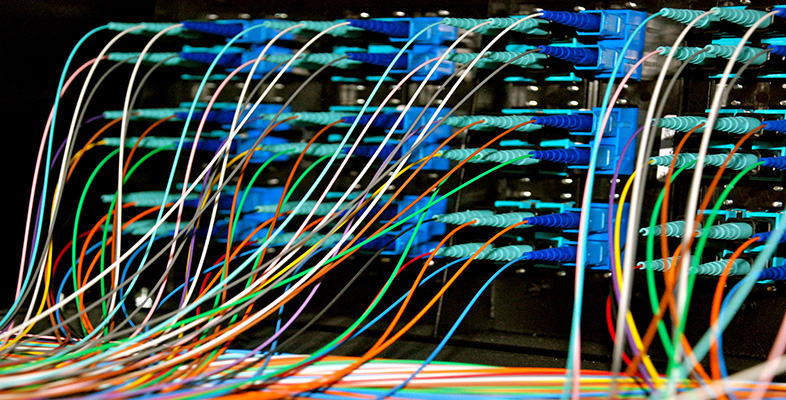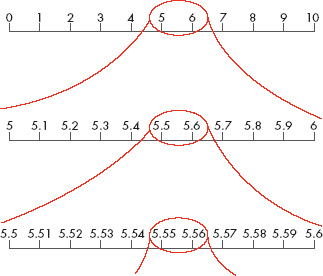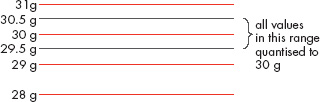Representing and manipulating data in computers

Start this free course now. Just create an account and sign in. Enrol and complete the course for a free statement of participation or digital badge if available.

Free course

# 2.5 Representing weights

A physical quantity such as weight has the property that it can take on any value, not just a finite set of values. For instance, at one time the ingredients in the scalepan could weigh 29.2569427 grams, at another time 125.1234659 grams, at yet another 2805.87625922 grams. It may not be possible for the scales to display such values, but they are physically possible. Quantities like weight whose values can take on any value in this way are said to be analogue.

Figure 3 may help to make this clearer for you, as it is a diagrammatic representation of an analogue quantity. At the top it shows a number line with gradations from 1 to 10. You can think of this as an analogue quantity that can vary in value between 0 and 10. Numbers between, say, 5 and 6 (such as 5.1 or 5.9) exist on the number line at the top of Figure 3, though they have not been explicitly picked out. You can see they exist if you look at the magnified number line between 5 and 6, where the gradations explicitly show 5.1, 5.2, etc. But numbers between, say, 5.5 and 5.6 also exist on this line, though they have not been explicitly picked out, as you can see on the even-more-magnified number line at the bottom of Figure 3. And this process could go on indefinitely, using greater and greater magnifications to see finer and finer distinctions. There are no breaks or gaps in the line that will be revealed by some increased magnification. And this is exactly what is true of an analogue quantity: there are no breaks or gaps in the values it can take.Figure 3 A diagrammatic representation of an analogue quantity

Figure 3 also serves to show the problem that arises when an analogue quantity is to be represented digitally, because the digital representation is rather like the markers along the lines of Figure 3: it can only take one of a set of finite values. If in a particular digital representation only the values 0, 0.1, 0.2, 0.3, … 1.0, 1.1, 1.2, … up to 10.0 are possible then an analogue value such as 5.53 (see the bottom line in Figure 3), which lies between 5.5 and 5.6, will have to be represented digitally by either 5.5 or 5.6. (In practice, 5.5 would be used as it is closer.)

Returning to the example of the kitchen scales, the fact that weight is an analogue quantity presents the difficulty I have just discussed when it is to be represented digitally: its value cannot be represented exactly. So a design decision has to be taken as to how exact the weight's representation is to be (to the nearest 10 grams? to the nearest gram? to the nearest 0.1 grams?), and a number of bits has to be allocated accordingly.

As you have already seen, in metric mode the scales weigh to the nearest gram. Hence they represent 29.2569427 grams as 29 grams, 125.1234659 as 125 grams and 2805.87625922 grams as 2806 grams. The infinitely large number of possible values between 0 and 3000 grams has been cut down to just 3001 values – the integer numbers of grams between 0 and 3000 inclusive.

The process of segmenting an analogue quantity such as weight into a finite number of values is known as quantisation, and the gap between consecutive values is known as the quantisation interval. (In the case of the scales, the quantisation interval is 1 gram.) The difference between the exact weight to be represented and the nearest of the finite number of values which can represent it is known as the quantisation error. In the above example of the scales, the largest possible quantisation error is ±0.5 grams because no actual weight value is more than half a gram from an integer number of grams. Figure 4 illustrates the idea of quantisation. The red lines represent the digital values that the scales work with. They are integer numbers of grams, and you can see that the quantisation interval is 1 gram. Any value from 29.5 grams up to just under 30.5 grams will be quantised to 30 grams, so the maximum quantisation error is ±0.5 grams.Figure 4 The quantisation interval is 1 gram and the largest possible quantisation error is ±0.5 grams

## Box 4: Reducing the quantisation error

The quantisation error can be made smaller by using a larger number of bits to represent values of the analogue quantity.

You already know that, because the kitchen scales are to weigh up to 3000 g to the nearest gram, 3001 different (positive) weight values are to be represented in the computer and 12 bits are needed to represent each possible value. But suppose the manufacturers had decided to represent weights to the nearest 0.1 gram instead of the nearest gram. Then there would be around ten times as many possible (positive) weight values to represent: 30 001, to be exact. Now, fifteen bits are needed to hold 30 001 different values – that's 3 extra bits per value. So the quantisation error has been reduced by reducing the quantisation interval. This means that the weight is being represented more closely, but at the expense of using more bits to hold the binary code.

Whether this is worthwhile in any particular situation will depend on why the values are being recorded and what they will be used for. In the case of domestic kitchen scales it is unlikely that recipes will call for less than whole numbers of grams, but in a delicate laboratory experiment it may be necessary to measure and record values not just to the nearest 0.1 gram but to the nearest 0.001 gram.

A further complication with representing weights arises from the fact that the weight in the scalepan may be changing. Some of the changes are sudden and isolated, for example when the user places a slab of butter into the scales to weigh it. Other changes may be ongoing, for example when the user slowly adds flour to the scalepan until a required weight has been achieved. Clearly it is necessary to take measurements sufficiently often, and to represent each measurement separately. Measurements taken at intervals are called samples. In order to decide how often samples need to be taken (another design decision), it is necessary to know how rapidly the weight is likely to be varying and also what the user will feel comfortable with. For the kitchen scales, probably every tenth of a second or so would be an appropriate rate at which to sample. In some industrial situations it may be necessary to sample much more frequently – or it may be adequate to sample much less frequently.

Although I have focused on representing weights in this section, what I have said is also applicable to a whole range of other physical quantities: for example, temperature, length, pressure, etc. Bear this in mind as you try Activity 14.

## Activity 14 (Self assessment)

A particular computer is designed to record the temperature of an oven in an industrial process to the nearest 0.1 °C. The temperature can vary from 200 °C to 250 °C.

1. What is the quantisation interval?

2. How many different finite values could a temperature sample take, and how many bits are needed to represent this number of finite values?

3. What is the largest possible quantisation error?

1. The quantisation interval is 0.1 °C.

2. The temperature values (in °C) could be 200.0, 200.1, 200.2, etc., up to 250.0. This is 501 possible values. Eight bits can only hold 256 different values, so 8 bits are not enough. But 9 bits can hold 2 × 256 = 512 bits, so 9 bits would be sufficient.

3. The maximum possible quantisation error will occur when the actual temperature is exactly halfway between two possible values, e.g. 200.05 °C. So it is ±0.05 °C.